# YOLOv5全面解析教程④：目標檢測模型精確度評估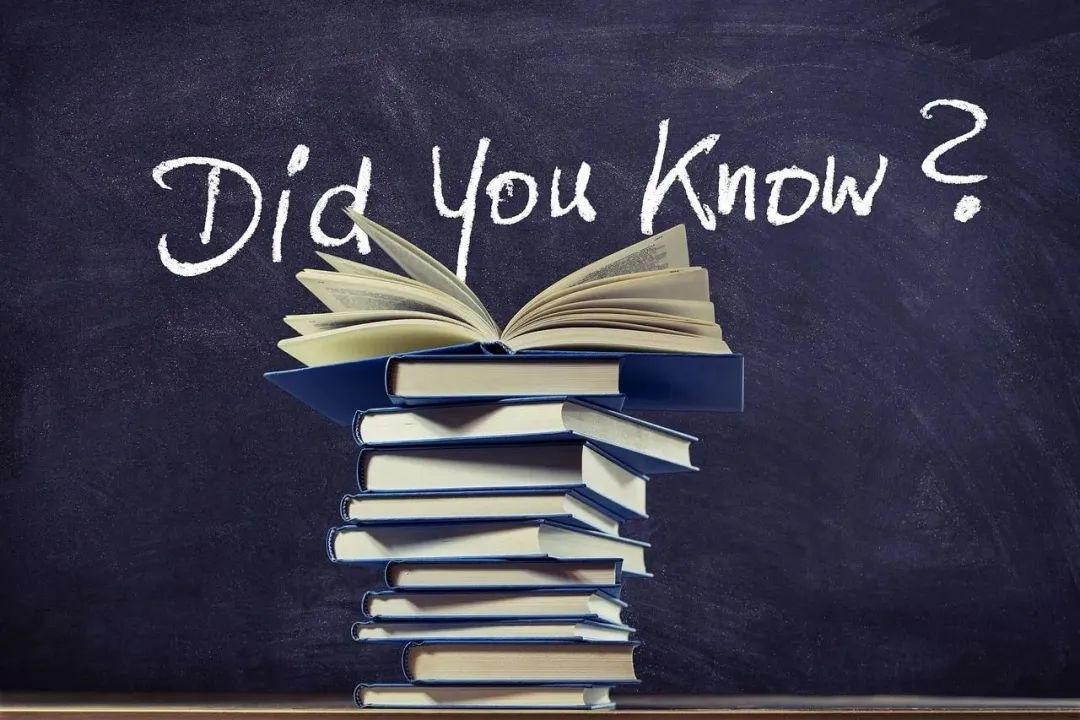BBuf

https://github.com/Oneflow-Inc/one-yolov5

## 指標評估（一些重要的定義）

### IOU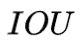(Intersection Over Union)  基於Jaccard索引，用於評估兩個邊界框之間的重疊程度。它需要一個真實迴歸框 (a ground truth bounding box)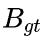和一個預測迴歸框(a predicted bounding box)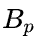計算得到。通過應用 IOU 我們能夠判斷出預測結果是有效（True Positive） 或者無效（False Positive）。也稱重疊度表示計算預測迴歸框和真實迴歸框的交併比,計算公式如下: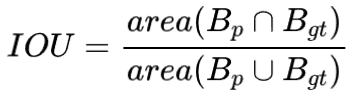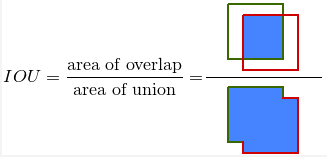### TP&FP&FN&TN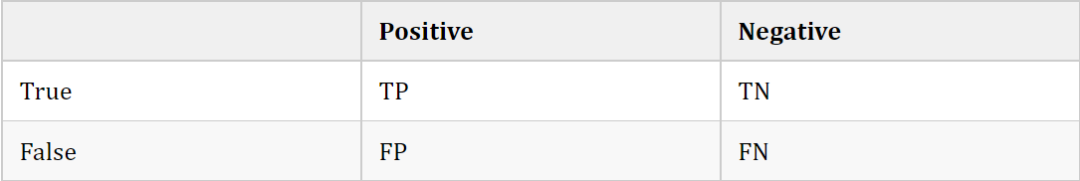• TP（True Postives）：分類器把正例正確的分類-預測為正例。(IOU >=  閾值)

• FN（False Negatives）：分類器把正例錯誤的分類-預測為負例。(IOU <  閾值)

• FP（False Postives）：分類器把負例錯誤的分類-預測為正例

• TN（True Negatives）：分類器把負例正確的分類-預測為負例（YOLOv5中沒有應用到

YOLOv5中沒有應用TN的原因: TN代表的是所有可能的未正確檢測到的邊界框。然而在YOLO在目標檢測任務中，每個網格會生成很多的預測邊界框，有許多的預測邊界框是沒有相應的真實標籤框，導致未正確檢測到的邊界框數量遠遠大於正確檢測到的邊界框，這就是為什麼不使用TN的原因。

threshold:  depending on the metric, it is usually set to 50%, 75% or 95%.

### Precision

Precision 定義：模型識別相關目標的能力。分類正確的樣本在所有樣本中的數量比例，公式如下: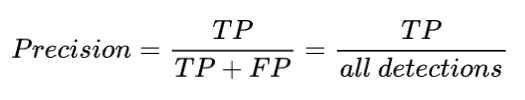### Recall

Recall 定義：是模型找到真實迴歸框(即標籤標註的框)的能力。計算公式如下：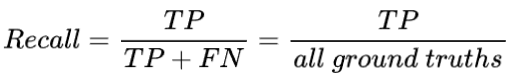### mAP

Confidence（置信度）：在統計學中，一個概率樣本的置信區間（Confidence interval）是對這個樣本的某個總體引數的區間估計。置信區間展現的是這個引數的真實值有一定概率落在測量結果的周圍的程度。置信區間給出的是被測量引數測量值的可信程度範圍，即前面所要求的“一定概率”。這個概率也被稱為置信水平。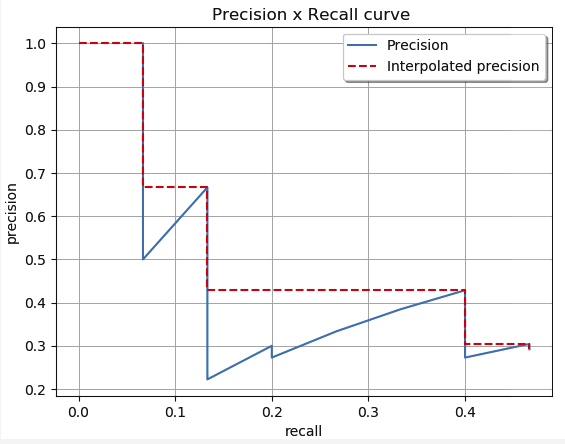(紅色曲線代表,人為的方式將PR曲線變成單調遞減，使得計算面積更容易。)

• AP（Average Percision）：AP為平均精度，指的是所有圖片內的具體某一類的PR曲線下的面積(橫軸為Recall，縱軸為Precision)。

• AP衡量的是對一個類檢測好壞，mAP就是對多個類的檢測好壞。在多類多目標檢測中，計算出每個類別的AP後，再除於類別總數，即所有類別AP的平均值，比如有兩類，類A的AP值是0.5，類B的AP值是0.2，那麼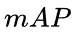=（0.5+0.2）/2=0.35。

• MAP：是指所有圖片內的所有類別的AP的平均值,map越高代表模型預測精度值越高。

•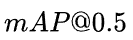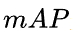是用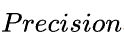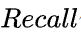作為兩軸作圖後圍成的面積，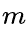表示平均，@後面的數表示判定正負樣本的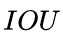閾值，其中@0.5表示IOU閾值取0.5。

•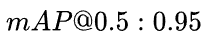：只以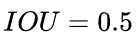的閥值的時候不一定就是好的模型，可能僅僅在0.5閥值表現得很好，在0.6,0.7...閥值表現得很差，為了更好地評估整體模型的準確度，因此計算一個模型在各個IOU值的AP(mAP)取平均值。

## 目標檢測中的mAP計算

### yolov5計算IOU原始碼解析

https://github.com/Oneflow-Inc/one-yolov5/blob/main/utils/metrics.py#L224-L261

``````# 計算兩框的特定iou (DIou, DIou, CIou)
def bbox_iou(box1, box2, xywh=True, GIoU=False, DIoU=False, CIoU=False, eps=1e-7):
# Returns Intersection over Union (IoU) of box1(1,4) to box2(n,4)

# Get the coordinates of bounding boxes 下面條件語句作用是:進行座標轉換從而獲取yolo格式邊界框的座標
if xywh:  # transform from xywh to xyxy
(x1, y1, w1, h1), (x2, y2, w2, h2) = box1.chunk(4, 1), box2.chunk(4, 1)
w1_, h1_, w2_, h2_ = w1 / 2, h1 / 2, w2 / 2, h2 / 2
b1_x1, b1_x2, b1_y1, b1_y2 = x1 - w1_, x1 + w1_, y1 - h1_, y1 + h1_
b2_x1, b2_x2, b2_y1, b2_y2 = x2 - w2_, x2 + w2_, y2 - h2_, y2 + h2_
else:  # x1, y1, x2, y2 = box1
b1_x1, b1_y1, b1_x2, b1_y2 = box1.chunk(4, 1)
b2_x1, b2_y1, b2_x2, b2_y2 = box2.chunk(4, 1)
w1, h1 = b1_x2 - b1_x1, b1_y2 - b1_y1
w2, h2 = b2_x2 - b2_x1, b2_y2 - b2_y1

# Intersection area 獲取兩個框相交的面積。
"""
left_line = max(b1_x1, b2_x1)
reft_line = min(b1_x2, b2_x2)
top_line = max(b1_y1, b2_y1)
bottom_line = min(b1_y2, b2_y2)
intersect = (reight_line - left_line) * (bottom_line - top_line)
"""
inter = (flow.min(b1_x2, b2_x2) - flow.max(b1_x1, b2_x1)).clamp(0) * \
(flow.min(b1_y2, b2_y2) - flow.max(b1_y1, b2_y1)).clamp(0)

# Union Area  兩個框併到面積
union = w1 * h1 + w2 * h2 - inter + eps

# IoU
iou = inter / union
if CIoU or DIoU or GIoU:
cw = flow.max(b1_x2, b2_x2) - flow.min(b1_x1, b2_x1)  # convex (smallest enclosing box) width
ch = flow.max(b1_y2, b2_y2) - flow.min(b1_y1, b2_y1)  # convex height
if CIoU or DIoU:  # Distance or Complete IoU https://arxiv.org/abs/1911.08287v1
c2 = cw ** 2 + ch ** 2 + eps  # convex diagonal squared
rho2 = ((b2_x1 + b2_x2 - b1_x1 - b1_x2) ** 2 + (b2_y1 + b2_y2 - b1_y1 - b1_y2) ** 2) / 4  # center dist ** 2
if CIoU:  # https://github.com/Zzh-tju/DIoU-SSD-pyflow.blob/master/utils/box/box_utils.py#L47
v = (4 / math.pi ** 2) * flow.pow(flow.atan(w2 / (h2 + eps)) - flow.atan(w1 / (h1 + eps)), 2)
alpha = v / (v - iou + (1 + eps))
return iou - (rho2 / c2 + v * alpha)  # CIoU
return iou - rho2 / c2  # DIoU
c_area = cw * ch + eps  # convex area
return iou - (c_area - union) / c_area  # GIoU https://arxiv.org/pdf/1902.09630.pdf
return iou  # IoU``````

### YOLOv5計算AP原始碼逐行解析

https://github.com/Oneflow-Inc/one-yolov5/blob/main/utils/metrics.py#L96-L121

``````# 根據PR曲線計算AP
def compute_ap(recall, precision):
""" Compute the average precision, given the recall and precision curves
# Arguments
recall:    The recall curve (list)
precision: The precision curve (list)
# Returns
Average precision, precision curve, recall curve
"""

# Append sentinel values to beginning and end 將開區間給補上，補成閉合的區間。
mrec = np.concatenate(([0.0], recall, [1.0]))
mpre = np.concatenate(([1.0], precision, [0.0]))

# Compute the precision envelope
"""
人為的把PR曲線變成單調遞減的,例如:
np.maximum(accumulate(np.array([21, 23, 18, 19, 20, 13, 12, 11]) ) => np.array([23, 23, 20, 20, 20, 13, 12, 11])
"""
mpre = np.flip(np.maximum.accumulate(np.flip(mpre)))

# Integrate area under curve
method = 'interp'  # methods: 'continuous', 'interp'
if method == 'interp': # 預設採用 interpolated-precision 曲線，
x = np.linspace(0, 1, 101)  # 101-point interp (COCO)
ap = np.trapz(np.interp(x, mrec, mpre), x)  # integrate
else:  # 'continuous'
i = np.where(mrec[1:] != mrec[:-1])  # points where x axis (recall) changes
ap = np.sum((mrec[i + 1] - mrec[i]) * mpre[i + 1])  # area under curve

return ap, mpre, mrec``````

## 參考文章